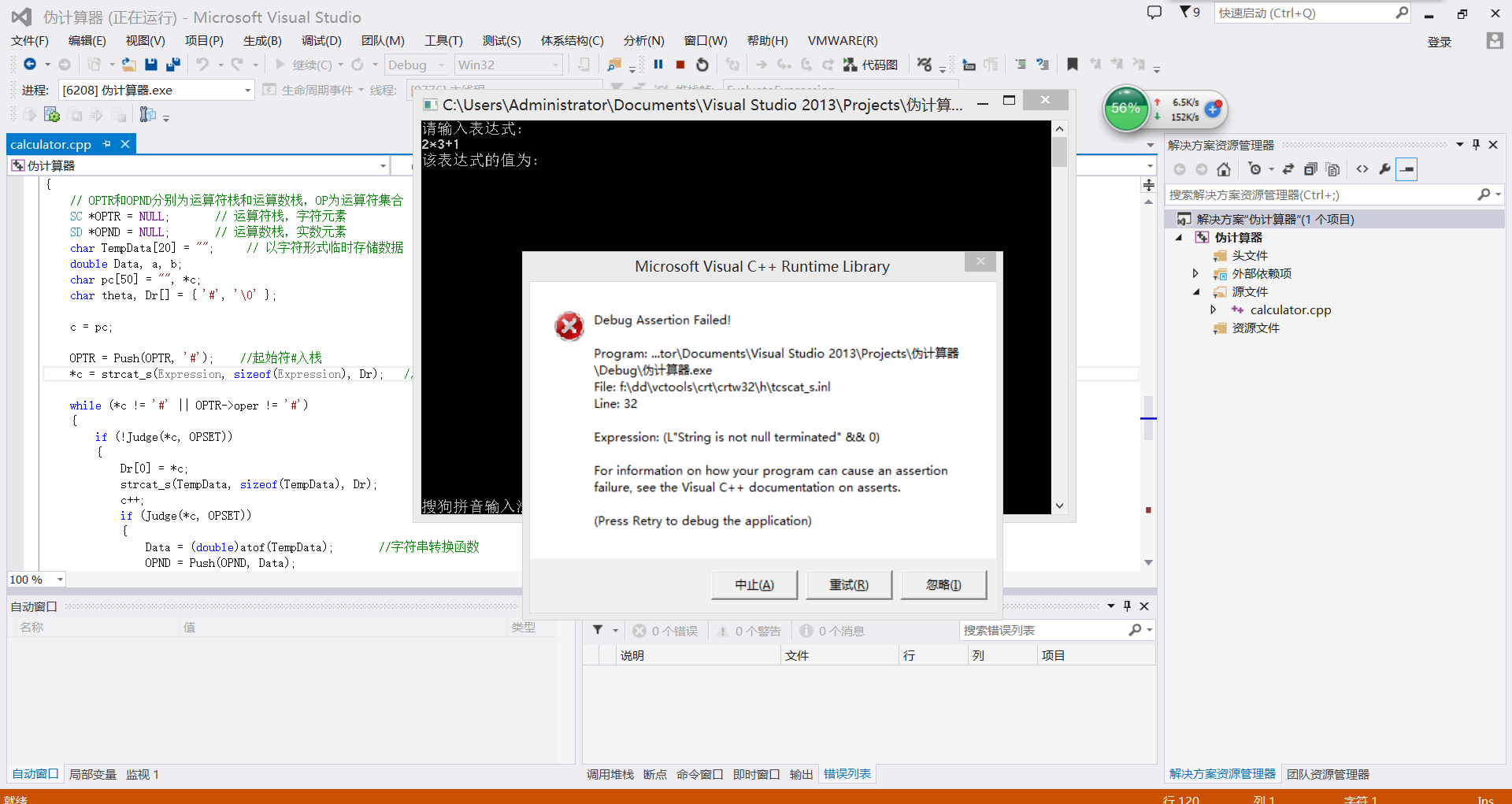C语言数据结构：表达式求值代码问题 20C#include
#include
#include
#include
#define true 1
#define false 0
#define OPSETSIZE 8 //运算符集合数为8

char OPSET[OPSETSIZE] = { '+', '-', '*', '/', '(', ')', '#', '^' };
unsigned char Prior =
{ /**********运算符优先级表***********/
// '+' '-' '*' '/' '(' ')' '#' '^'
/*'+'*/ '>', '>', '<', '<', '<', '>', '>', '<',
/*'-'*/ '>', '>', '<', '<', '<', '>', '>', '<',
/*'*'*/ '>', '>', '>', '>', '<', '>', '>', '<',
/*'/'*/ '>', '>', '>', '>', '<', '>', '>', '<',
/*'('*/ '<', '<', '<', '<', '<', '=', ' ', '<',
/*')'*/ '>', '>', '>', '>', ' ', '>', '>', '>',
/*'#'*/ '<', '<', '<', '<', '<', ' ', '=', '<',
/*'^'*/ '>', '>', '>', '>', '<', '>', '>', '>'
};

typedef int Status;
typedef struct StackChar
{
char oper;
struct StackChar next;
}SC; //StackChar类型的结点SC
typedef struct StackDouble
{
double num;
struct StackDouble *next;
}SD; //StackDouble类型的结点SD
/
********FUN:SC类型的指针入栈，返回p **********/
SC Push(SC *s, char oper)
{
SC *p = (SC
)malloc(sizeof(SC));
p->oper = oper;
p->next = s;
return p;
}
/***********FUN:SC类型的指针出栈**************/
SC Pop(SC *s)
{
SC *q = s;
s = s->next;
free(q);
return s;
}
/
*********FUN:SF类型的指针入栈，返回p********/
SD Push(SD *s, double num)
{
SD *p = (SD
)malloc(sizeof(SD));
p->num = num;
p->next = s;
return p;
}
/**************FUN:SF类型的指针出栈**********/
SD Pop(SD *s)
{
SD *q = s;
s = s->next;
free(q);
return s;
}
/
***************FUN:计算 aθb 的逻辑操作****************/
double Calculator(double a, unsigned char theta, double b)
{
switch (theta)
{
case '+': return a + b;
case '-': return a - b;
case '*': return a*b;
case '/': return a / b;
case '^': return pow(a, b);
default: return 0;
}
}
/***********Fun：判断输入运算符是否为8种标准运算符********/
Status Judge(char Test, char TestOp)
{
int i;
int Find = false;
for (i = 0; i< OPSETSIZE; i++)
{
if (Test == TestOp[i])
Find = true;
}
return Find;
}
/
*************Fun：返回输入运算符的设定数值**************/
Status ReturnOpOrd(char oper, char TestOp)
{
int i;
for (i = 0; i< OPSETSIZE; i++)
{
if (oper == TestOp[i])
return i;
}
}
/
*********FUN：比较运算符优先级**********/
char Precede(char Aop, char Bop)
{
return Prior[ReturnOpOrd(Aop, OPSET)][ReturnOpOrd(Bop, OPSET)];
}
/*************FUN:表达式求值*************/
double EvaluateExpression(char* Expression)
{
// OPTR和OPND分别为运算符栈和运算数栈，OP为运算符集合
SC *OPTR = NULL; // 运算符栈，字符元素
SD *OPND = NULL; // 运算数栈，实数元素
char TempData = ""; // 以字符形式临时存储数据
double Data, a, b;
char pc = "", *c;
char theta, Dr[] = { '#', '\0' };

``````c = pc;

OPTR = Push(OPTR, '#');    //起始符#入栈
*c = strcat_s(Expression, sizeof(Expression), Dr);   //表达式追加尾部#

while (*c != '#' || OPTR->oper != '#')
{
if (!Judge(*c, OPSET))
{
Dr = *c;
strcat_s(TempData, sizeof(TempData), Dr);           //字符串连接函数
c++;
if (Judge(*c, OPSET))
{
Data = (double)atof(TempData);       //字符串转换函数
OPND = Push(OPND, Data);
strcpy_s(TempData,sizeof(TempData)," \0");                         //清空临时存放数据的字符串
}
}
else    // 不是运算符则进栈
{
switch (Precede(OPTR->oper, *c))
{
case '<': // 栈顶元素优先级低
OPTR = Push(OPTR, *c);
c++;
break;
case '=': // 脱括号并接收下一字符
OPTR = Pop(OPTR);
c++;
break;
case '>': // 退栈并将运算结果入栈
theta = OPTR->oper;
OPTR = Pop(OPTR);
b = OPND->num;
OPND = Pop(OPND);
a = OPND->num;
OPND = Pop(OPND);
OPND = Push(OPND, Calculator(a, theta, b));
break;
}
}
}
return OPND->num;
``````

}

int main(void)
{
char s;
puts("请输入表达式:");
gets_s(s);
puts("该表达式的值为:");
printf("%s =%lf\n", s, EvaluateExpression(s));
system("pause"); //Press any key to exit
return 0;
}

2个回答

`````` /*经典算法-算术表达式求值

2 中缀表达式和后缀表达式

3 运算符的优先级

1 括号()
2 负号-
3 乘方**
4 乘*，除/，求余%
5 加+，减-
6 小于<，小于等于<=，大于>，大于等于>=
7 等于==，不等于!=
8 逻辑与&&
9 逻辑或||

*/
#include<iostream>
#include<string.h>
#include<stdlib.h>
using namespace std;
template <class T>
class stack
{
public:
stack(){top= -1;SIZE=2;buffer=new T[SIZE];}; //SIZE=2（过小）用于测试PUSH函数
~stack(){if(buffer) delete []buffer;};//析构函数
bool push(T element); //入栈
T& pop();//出栈
void clear(){ top=-1;}
private:
T *buffer;
int top;
int SIZE;
};
template <class T>
bool stack<T>::push(T element)
{
top++;
if (top>SIZE-1)
{
SIZE+=10;
T* temp  =new T [SIZE];
//  for(int i=0;i<SIZE-10;i++)
// temp[i]=buffer[i];//将对象 COPY
memcpy((void*)temp,(void*)buffer,(SIZE-10)*sizeof(T));//两种方法都可
delete buffer;
buffer=temp;
}//满
buffer[top]=element;
return true;
}
template <class T>
T& stack<T>::pop()
{
return buffer[top--];
}
bool IsOperand( char ch )
{
char operators[] = { '+', '-', '*', '/', '(', ')' };
for(int i=0; i<6; i++)
if( ch == operators[i] )
return false;
return true;
}
int Priority( char ch )
{
int priority;
switch( ch )
{
case '+' :
priority = 1;
break;
case '-' :
priority = 1;
break;
case '*' :
priority = 2;
break;
case '/' :
priority = 2;
break;
default :
priority = 0;
break;
}
return priority;
}
double GetValue(char op, double ch1, double ch2)
{
switch( op )
{
case '+':
return ch2 + ch1;
case '-':
return ch2 - ch1;
case '*':
return ch2 * ch1;
case '/':
return ch2 / ch1;
default:
return 0;
}
}
//将中缀表达式解析成后缀表达式
/*

（1）从右向左依次取得数据ch。
（2）如果ch是操作数，直接输出。
（3）如果ch是运算符（含左右括号），则：
a：如果ch = '('，放入堆栈。
b：如果ch = ')'，依次输出堆栈中的运算符，直到遇到'('为止。
c：如果ch不是')'或者'('，那么就和堆栈顶点位置的运算符top做优先级比较。
1：如果ch优先级比top高，那么将ch放入堆栈。
2：如果ch优先级低于或者等于top，那么输出top，然后将ch放入堆栈。
（4）如果表达式已经读取完成，而堆栈中还有运算符时，依次由顶端输出。

（1）读取'('，压入堆栈，output为空
（2）读取A，是运算数，直接输出到output字符串，output = A
（3）读取'-'，此时栈里面只有一个'('，因此将'-'压入栈，output = A
（4）读取B，是运算数，直接输出到output字符串，output = AB
（5）读取')'，这时候依次输出栈里面的运算符'-'，然后就是'('，直接弹出，output = AB-
（6）读取'*'，是运算符，由于此时栈为空，因此直接压入栈，output = AB-
（7）读取C，是运算数，直接输出到output字符串，output = AB-C
（8）读取'+'，是运算符，它的优先级比'*'低，那么弹出'*'，压入'+"，output = AB-C*
（9）读取D，是运算数，直接输出到output字符串，output = AB-C*D
（10）读取'-'，是运算符，和'+'的优先级一样，因此弹出'+'，然后压入'-'，output = AB-C*D+
（11）读取E，是运算数，直接输出到output字符串，output = AB-C*D+E
（12）读取'/'，是运算符，比'-'的优先级高，因此压入栈，output = AB-C*D+E
（13）读取F，是运算数，直接输出到output字符串，output = AB-C*D+EF
（14）原始字符串已经读取完毕，将栈里面剩余的运算符依次弹出，output = AB-C*D+EF/-
*/
char* Parse(const char *expr)
{
//  const char *exp = expr;
int i, j = 0;
char ch, ch1;
const char* A = expr;
char *B  = new char[strlen(expr)+1]; //最后生成的后缀表达式
stack<char>  myStack;
for(i=0;ch = A[i]; i++)///A[I]='
``````